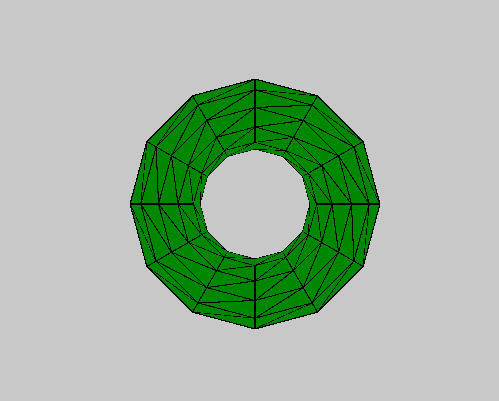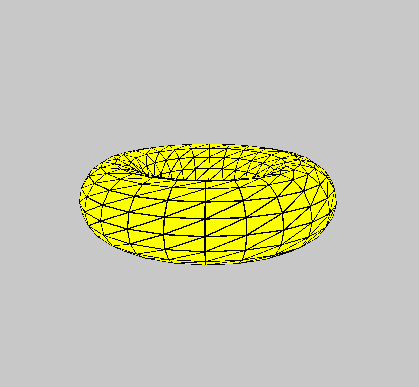Related Articles

# p5.js | torus() Function

• Last Updated : 22 Apr, 2019

The torus() function in p5.js is used to draw a torus with given torus radius and tube radius.

Syntax:

`torus( radius, tubeRadius, detailX, detailY )`

Parameters: This function accepts four parameters as mentioned above and described below:

• detailX: This parameter stores the number of segments in x-dimension.
• detailY: This parameter stores the number of segments in y-dimension.

Below programs illustrate the torus() function in p5.js:

Example 1: This example uses torus() function to draw a torus with given torus radius and tube radius.

 `function` `setup() {``     ` `    ``// Create Canvas of size 600*600``    ``createCanvas(600, 600, WEBGL);``}``  ` `function` `draw() {``     ` `    ``// Set background color``    ``background(200);``    ` `    ``// Set fill color of torus``    ``fill(``'green'``);``    ` `    ``// Call to torus function``    ``torus(90, 35, 12, 12);``}`

Output:Example 2: This example uses torus() function to draw a torus with given torus radius and tube radius.

 `function` `setup() {``     ` `    ``// Create Canvas of size 600*600``    ``createCanvas(600, 600, WEBGL);``}``  ` `function` `draw() {``     ` `    ``// Set background color``    ``background(200);``    ` `    ``// Set fill color of torus``    ``fill(``'yellow'``);``    ` `    ``// Rotate ``    ``rotateX(frameCount * 0.01);``    ``rotate(frameCount*0.05);``    ` `    ``// Call to torus function``    ``torus(90, 35);``}`

Output:Reference: https://p5js.org/reference/#/p5/torus

Hey geek! The constant emerging technologies in the world of web development always keeps the excitement for this subject through the roof. But before you tackle the big projects, we suggest you start by learning the basics. Kickstart your web development journey by learning JS concepts with our JavaScript Course. Now at it’s lowest price ever!

My Personal Notes arrow_drop_up